Journal of Measurements in Engineering

Published: 30 June 2019

# Comparison of different magneto-rheological fluids’ stability

Joris Vėžys1
Egidijus Dragašius2
Kazimieras Juzėnas3
Evguenia Korobko4
1, 2, 3Kaunas University of Technology, Studentu str. 56, Kaunas, Lithuania
4Heat and Mass Transfer Institute of NAS of Belarus, 15 P. Brovka str., 220072, Minsk, Belarus
5Bialystok University of Technology, Wiejska 45C, 15-351 Bialystok, Poland
Corresponding Author:
Joris Vėžys
Views 193

#### Abstract

The purpose of this paper is to compare two different methods for describing the sedimentation in different MRFs. It was decided to obtain electric resistivity of the MR fluid under magnetic field and to measure inductance of the solenoid, which is around the MR fluid, for diagnostic of the sedimentation. The electrical resistivity of the MR fluids and inductivity of the solenoid was measured daily for 11 days for 5 different MR fluids: (Lord MRF-140CG, Lord MRF-122EG, Liquids Research Company MRHCCS4-A and Liquids Research Company MRHCCS4-B and MUDZH-3 made in A. V. Luykov Heat and Mass Transfer Institute, Minsk, BY). These two methods and according procedures are described below.

## 1. Introduction

A magneto-rheological fluid (MR fluid or MRF) is a type of smart fluid where micro sized particles are in a carrier fluid, usually a type of oil. When applied by a magnetic field, the fluid greatly increases its apparent viscosity, to the point of becoming a viscoelastic solid. Importantly, the yield stress of the fluid under magnetic field can be controlled very accurately in a few milliseconds by varying on magnetic field intensity.

One of the biggest problem of magneto-rheological fluids is their sedimentation. The sedimentation of particles in the fluid is very harmful when MR fluids are used in clutches, dampers, brakes, shock absorbers, bridges, prosthesis, etc. [1, 2] When sedimentation of particles occurs in MR fluid, working of the device can cause some errors or can be not stable. The decrease of the sedimentation rate can be achieved by adding surfactants to the MR fluid, or use a stabilizer to give plastic, thixotropic properties to the carrying fluid . The main thing for increasing stability of the MR fluid is to control the sedimentation rate. To determine sedimentation level in the MR fluid ultrasonic sensors can be used . Velocity of the ultrasonic sensors differs under magnetic field and when sedimentation occurs. Another method to identify the sedimentation level in MR fluid is to measure a temperature change in two sensors. One sensor is made to heat a fluid, and another is to measure the difference of the temperature. The more particle is settled, the more temperature change it can cause . Also, resistivity changes while magnetic field is applied were described in a literature [6, 7], but they do not describe how the sedimentation influences the electrical resistivity of MR fluid.

The aim of research was to compare two different methods of describing the sedimentation in different MRFs. It was decided to obtain electric resistivity of the MR fluid under magnetic field and to measure inductance of the solenoid, which is around the MR fluid, to diagnose the rate of the sedimentation.

The MR fluids’ electric resistivity and inductivity of the solenoid was measured daily for 11 days for 5 different MR fluids: (Lord MRF-140CG, Lord MRF-122EG, Liquids Research Company MRHCCS4-A and Liquids Research Company MRHCCS4-B and MUDZH-3, made in A. V. Luikov Heat and Mass Transfer Institute, Minsk, BY). These two methods and according procedures are described below.

## 2. Theoretical base

The measurement of the induction of a solenoid, which is about the MR fluid  can be named as a way to determine the sedimentation. In this way, the inductance of a solenoid can be given in this equation:

1
$L=\frac{{N}^{2}{\mu }_{0}\mu A}{l}={L}_{0}\mu .$

As it is shown in the equation, $N$ is for the number of turns; ${\mu }_{0}$ is for the permeability of free space (a vacuum) and it is a physical constant equal to approximately 1.2563×10-6 [H/m]; $\mu$ is for the relative magnetic permeability of a sample which enveloped by a solenoid [H/m]; $A$ is the cross section of a solenoid; l is chosen to illustrate the solenoid length; and ${L}_{0}$ is for the inductance of a solenoid in a vacuum. From Eq. (1) the sensitivity of the solenoid inductance to the magnetic properties of a medium inside it can been noticed.

Furthermore, in 2013, Xi Chen  has created the theoretical model of resistance. This model is based on the theory, that ferromagnetic particles and particles of a liquid are replaced by a small equal size resistor and that way we can determine a resistivity of the MR fluid when changeable magnetic field is applied on it. So, the whole MR fluid can be equaled to the resistors connected by a series-parallel circuit. Let’s say that all the particles are of uniform size their diameter are d and the resistance of each Fe3O4 particle is ${r}_{1}$ and ${r}_{2}$ stands for the resistance of the carrier liquid particles . A scheme is given in a Fig. 1. The same idea is used in another work, where particles are equaled to the resistors .

Fig. 1A scheme where ferromagnetic and carrier liquid particles are equaled to similar size resistors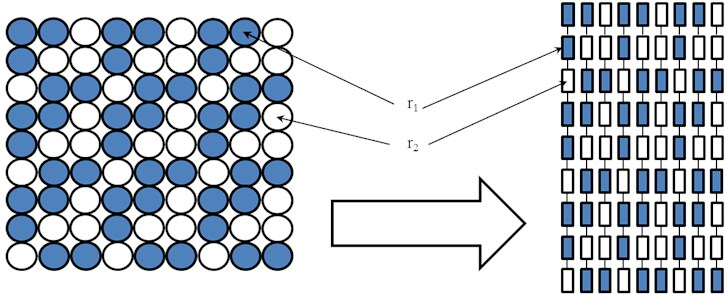The magnetization process  of MR fluid can be classified into four stages (Fig. 2). In stage one, the ferromagnetic particles freely distribute in the oil media, the sample is non-conducting. In stage two, the particles become polarized under the magnetic field and some chains form columns with some uncompleted chains and clusters. It causes the sample’s resistance to decrease sharply. In stage three, the uncompleted chains and clusters formed in stage two form a new chains and all the chains aggregate into a column, the whole resistance continues to decrease. In stage four, the residual particles join to the column, the resistance decreases very slowly with the increasing magnetic field until it is saturated.

Fig. 2The magnetization process of MR fluid can be classified into four stages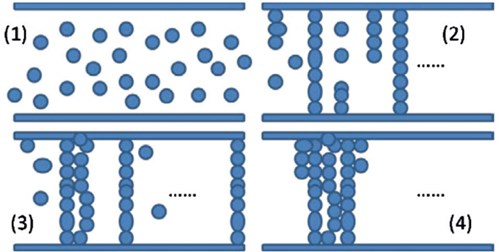## 3. Experimental setups

The experimental setup for measuring electrical resistivity in MR fluids is shown in Fig. 3.

Fig. 3Experimental setup No. 1: 1 – electric coils, 2 – digital ohmmeter, 3 – tube filled with MR fluid, 4 – sensor to measure the electrical resistivity, 5 – external power supply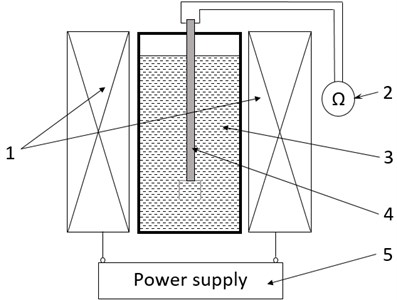A tube with magneto-rheological fluid 3 was putted to the electrical coil powered by the power supply 5, to obtain magnetic field. Special made resistivity measurement sensor 4 was placed inside the tube with MR fluid. This sensor was connected to the digital ohmmeter 2.

The sensor consists of two copper plates, which are separated by one millimeter gap and are attached to the headstock. The inner side of plates was electrical conductible. Resistance of magneto-rheological fluid was measured using multimeter PeakTech 2005. The range of resistance measuring for this device is 0,1 Ω-2 GΩ.

The scheme of the experimental setup for measuring inductance of a solenoid determining the sedimentation of MR fluids is shown in Fig. 4.

Fig. 4 illustrates the working principle of the inductance measurement device. MR fluid in the glass tube is placed inside the solenoid. The top of the fluid sample is aligned to the start of the turns of the solenoid as it is shown in Fig. 4. Because of settling of the particles, only a small amount of them are in the upper layer, so there is probably only carrier fluid there. There is a mudline which separates settling MR fluid and only carrier fluid. On the bottom part of the tube there are settled particles.

The sedimentation stability of five different magneto-rheological fluids (Lord MRF-140CG, Lord MRF-122EG, Liquids Research Company MRHCCS4-A and Liquids Research Company MRHCCS4-B and MUDZH-3, made in A. V. Luikov Heat and Mass Transfer Institute, Minsk, BY) was determined.

The concentration of particles in different MR fluids are given here: MRF-140CG – 85 %, MRF-122EG – 72 %, MRHCCS4-A – 70 %, MRHCCS4-B – 80 %, MUDZH-3 – 71 %.

Fig. 4Experimental setup No.2: MR fluid is in a glass tube which is placed inside the solenoid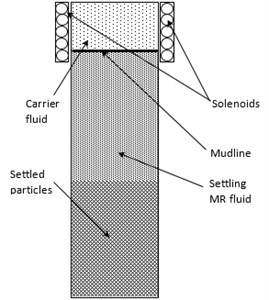## 4. Experimental results

The resistivity was measured daily for 11 days using resistivity measuring method, when magnetic field strength of 80 kA/m for all five MR fluids was applied. Results are shown in Fig. 5.

Fig. 5Electrical resistivity using magnetic field strength of 80 kA/m determined in 11 days for all five MR fluids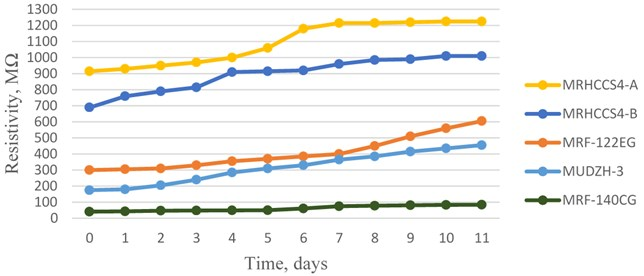The highest concentration of particles MR fluid MRF-140CG obtained lowest electrical resistivity during the time (40-90 MΩ). MRF-122EG and MUDZH-3 MR fluids have probably the same concentration of particles and their measured electrical resistivity also was nearly the same. Fluids, which is made by Liquids research company, MRHCCS4-A and MRHCCS4-B, have higher electrical resistivity compared to other MR fluids. Also, higher resistivity is in the fluid MRHCCS4-A, which has smaller concentration of particles than MRHCCS4-B.

Also, solenoid inductance measuring method was used to determine the sedimentation level in all five MR fluids. The difference between zero inductivity (inductivity of mixed fluid) and measured values of inductivity were calculated and named $∆L$. The results are shown in Fig. 6.

The MRF-122EG and MUDZH-3 have higher inductivity change than other compared fluids. Liquids Research co. fluids has nearly the same inductivity change, but using this method determined, that sedimentation level is a little bit higher for the MRHCCS4-B fluid. Also, fluid MRF-140CG has a higher inductivity change than Liquids Research co. fluids, but less than MRF-122EG and MUDZH-3. The inductivity changes for both LORD fluids is quite different. The same manufacturer should add same additives to maintain sedimentation level as low as possible, but this could maybe happen because of different concentration of particles (72 % and 85 %).

Resistivity measuring method is better compared to inductance measuring method because it is simpler to install a experimental setup and more accurate results can be obtained by this method, because resistance can be measured in different levels (different heights) of measuring MR fluid. It also has some disadvantages. One of them is that resistivity of MR fluid when it is under magnetic field is quite big (up to 1 GΩ), so laboratory equipment is needed to measure such a big electrical resistivity.

Fig. 6Inductivity difference dependence on sedimentation time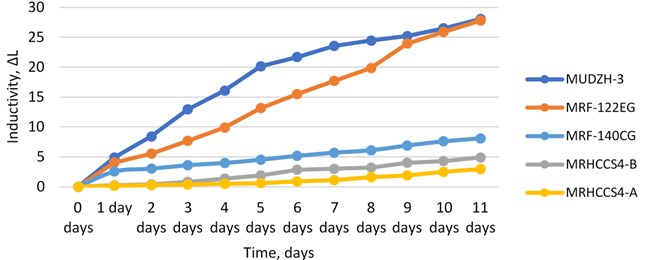## 5. Conclusions

Five different type of MR fluids were tested using two different methods to determine sedimentation in these fluids. Measurements were performed daily for 11 days. Using electrical resistivity measuring method results obtained by magnetic field strength of 80 kA/m. The highest concentration of particles MR fluid MRF-140CG obtained lowest electrical resistivity during the time (40-90 MΩ). MRF-122EG and MUDZH-3 MR fluids measured electrical resistivity also was nearly the same after 5 days. The other days electrical resistivity of MRF-122EG is higher. MRHCCS4-A and MRHCCS4-B, have higher electrical resistivity compared to other MR fluids (700-1300 MΩ). A difference between Liquid Research co. fluids and others may be the additives added to these fluids or their particles have less electric conductivity than others. The lowest sedimentation percentially is for Liquids Research co. fluids. Its 4 times less than LORD fluids, and 6 times less than MUDZH-3. Using inductance-measuring method the inductance of solenoid was measured. To simplify the results difference between firstly measured (zero inductivity) and inductivity measured after a period time were calculated and named $∆L$. MUDZH-3 and MRF-122EG have higher inductivity than other three MR fluids. This phenomenon is seen by both methods, so we can say that fluids MRF-122EG and MUDZH-3 have probably the same level of sedimentation. Other three fluids have a small inductivity change (lower than 10). As it is in resistivity measuring method, Liquid Research co. fluids have nearly the same sedimentation level. The only fluid, which results is not the same in both methods is MRF-140CG. When using resistivity measuring method this fluid had lowest resistivity comparing to other fluids, but using inductance measuring method its inductivity was higher than Liquid Research co. fluids.

Also, correlation coefficients for every fluid measurement by different method were obtained. The correlation coefficient is a statistical measuring method which calculates the strength of the relationship between the relative movements of the two variables. The coefficient of 1 shows a perfect positive correlation. Positive correlation is a relationship between two variables in which both variables move in tandem. Here are the values for tested MR fluids: MRF-140CG – 0,92, MRF-122EG – 0,93, MRHCCS4-A – 0,95, MRHCCS4-A – 0,89, MUDZH-3 – 0,98. So we can clearly compare these two methods to determine sedimentation in MR fluids. Both methods are reliable and determine the level of sedimentation great, but electrical resistivity method is quite simple and can achieve more accurate results than inductivity measuring method.